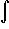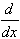#Interactive Real Analysis

Next | Previous | Glossary | Map

## 7.2. Integration Techniques

### Example 7.2.6(d): Applying Integration by Parts

Findsin5(x) dx
As in the previous example, it seems that integration by parts does not apply because there is no product. But we can write sin5(x) = sin(x) sin4(x) and define the functions
• g'(x) = sin(x) so that g(x) = -cos(x)
• f(x) = sin4(x) so that f'(x) = 4 sin(x)3 cos(x)
Then G(x) = f(x) g(x) = -cos(x) sin4(x) andsin5(x) dx = -cos(x) sin4(x) + 4sin(x)3 cos2(x) dx =
= -cos(x) sin4(x) + 4sin(x)3 (1 - sin2(x)) dx =
= -cos(x) sin4(x) + 4sin(x)3 dx - 4sin5(x) dx
It seems as if we are stuck. Not only did we get the original integralsin5(x) dx back, but we introducedsin3(x) dx which seems just about as complicated as the original integral. However, with a little more abstraction we can solve the original problem "by magic".

Let

Ik =sink(x) dx =sin(x) sink-1(x) dx
Define the functions
• g'(x) = sin(x) so that g(x) = -cos(x)
• f(x) = sink-1(x) so that f'(x) = (k-1) cos(x) sink-2(x)
Then
Ik = -cos(x) sink-1(x) + (k-1)cos2 sink-2(x) dx =
= -cos(x) sink-1(x) + (k-1)(1 - sin2) sink-2(x) dx =
= -cos(x) sink-1(x) + (k-1)sink-2(x) dx - (k-1)sink(x) dx =
= -cos(x) sink-1(x) + (k-1) Ik-2 - (k-1) Ik
Solving this expression for Ik we get
Ik = -1/k cos(x) sink-1(x) + (k-1)/k Ik-2
But this is a recursive formula for computing Ik for any integer k. In particular we have
• I0 =sin0(x) dx =1 dx = x
• I1 =sin1(x) dx = -cos(x)
• I2 = -1/2 cos(x) sin(x) + 1/2 I0 = -1/2 cos(x) sin(x) + 1/2 x
• I3 = -1/3 cos(x) sin2(x) + 2/3 I1 = -1/3 cos(x) sin2(x) - 2/3 cos(x)
• I4 = -1/4 cos(x) sin3(x) + 3/4 I2 =
= -1/4 cos(x) sin3 + 3/4 (-1/2 cos(x) sin(x) + 1/2 x)
• I5 = -1/5 cos(x) sin4(x) + 4/5 I3 =
= -1/5 cos(x) sin4(x) + 4/5 (-1/3 cos(x) sin2(x) - 2/3 cos(x))
Indeed, a - cumbersome - check would show thatI5 = sin5, so that our recursive formula and the resulting answer for our particular example seem correct.
Next | Previous | Glossary | Map